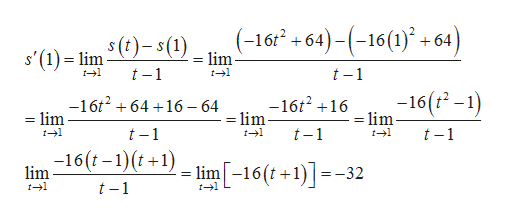A rock is dropped from a height of 64ft. Its height above ground at time t seconds later is given by s(t)=−16t2+64 for 0≤t≤2. Find its instantaneous velocity 1 second after it is dropped using the limit definition of a derivative. Do not include units in your answer.

Question

A rock is dropped from a height of 64ft. Its height above ground at time t seconds later is given by s(t)=−16t2+64 for 0≤t≤2. Find its instantaneous velocity 1 second after it is dropped using the limit definition of a derivative. Do not include units in your answer.

Step 1

Instantaneous velocity at t=1 is ds/dt at t=1 , that is s'(1).

Step 2

We substitute s(t) and s(1) in the lim...help_outlineImage Transcriptionclose(-16 +64)(-16(1) 64) s'(1) lim-(lim- t1 t 1 t-1 -16 (-1) -16t216 - lim -16t264 16 64 - lim = lim t-1 t-1 t-1 -16(t-1)(t+1) lim[-16(t+1)=- lim 32 t 1 fullscreen

Want to see the full answer?

See Solution

Want to see this answer and more?

Our solutions are written by experts, many with advanced degrees, and available 24/7

See Solution
Tagged in

Derivative